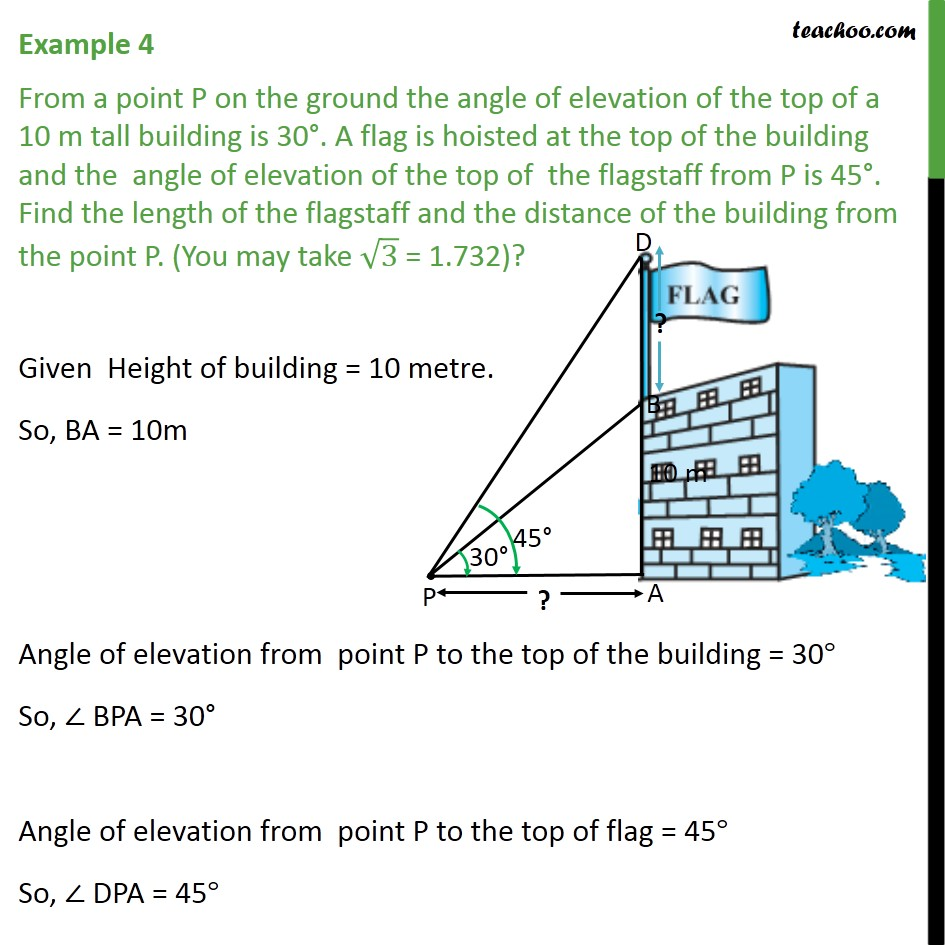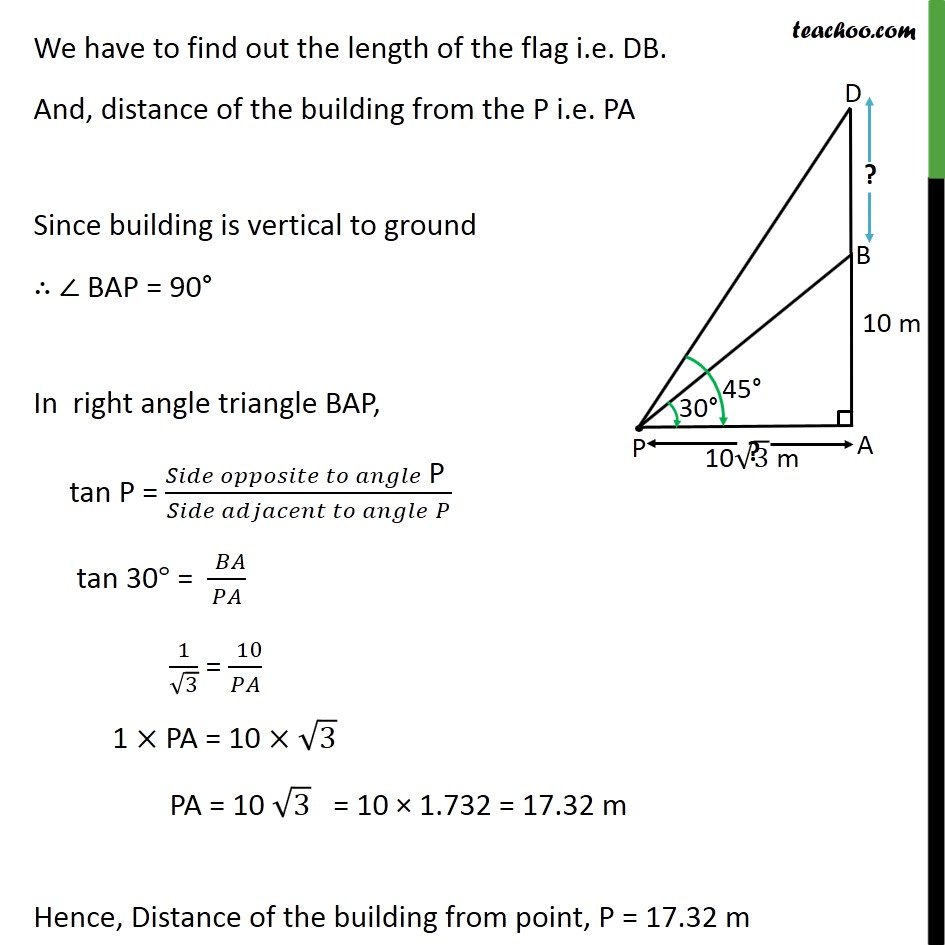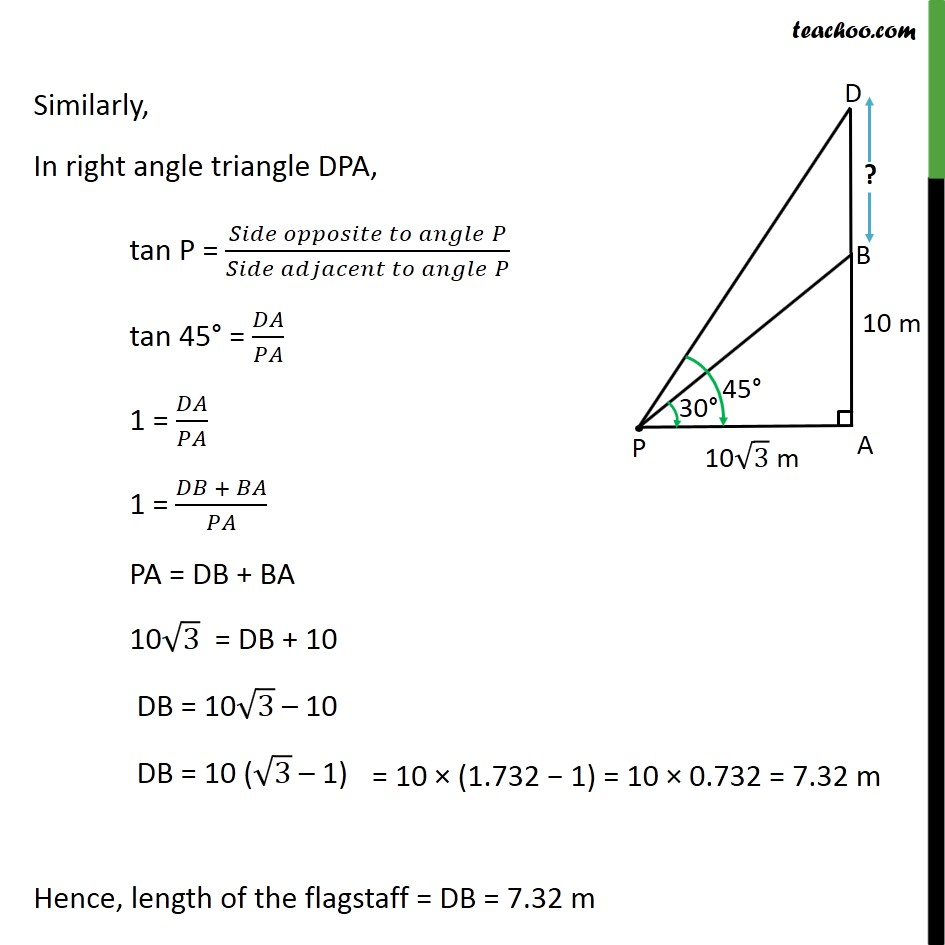1. Chapter 9 Class 10 Some Applications of Trigonometry
2. Concept wise
3. Questions easy to difficult

Transcript

Example 4 From a point P on the ground the angle of elevation of the top of a 10 m tall building is 30°. A flag is hoisted at the top of the building and the angle of elevation of the top of the flagstaff from P is 45°. Find the length of the flagstaff and the distance of the building from the point P. (You may take √3 = 1.732)? Given Height of building = 10 metre. So, BA = 10m Angle of elevation from point P to the top of the building = 30° So, ∠ BPA = 30° Angle of elevation from point P to the top of flag = 45° So, ∠ DPA = 45° We have to find out the length of the flag i.e. DB. And, distance of the building from the P i.e. PA Since building is vertical to ground ∴ ∠ BAP = 90° In right angle triangle BAP, tan P = (𝑆𝑖𝑑𝑒 𝑜𝑝𝑝𝑜𝑠𝑖𝑡𝑒 𝑡𝑜 𝑎𝑛𝑔𝑙𝑒" P " )/(𝑆𝑖𝑑𝑒 𝑎𝑑𝑗𝑎𝑐𝑒𝑛𝑡 𝑡𝑜 𝑎𝑛𝑔𝑙𝑒" " 𝑃) tan 30° = (" " 𝐵𝐴)/𝑃𝐴 1/√3 = (" " 10)/𝑃𝐴 1 × PA = 10 × √3 = 10 × 1.732 = 17.32 m PA = 10 √3 Hence, Distance of the building from point, P = 17.32 m Similarly, In right angle triangle DPA, tan P = (𝑆𝑖𝑑𝑒 𝑜𝑝𝑝𝑜𝑠𝑖𝑡𝑒 𝑡𝑜 𝑎𝑛𝑔𝑙𝑒" " 𝑃)/(𝑆𝑖𝑑𝑒 𝑎𝑑𝑗𝑎𝑐𝑒𝑛𝑡 𝑡𝑜 𝑎𝑛𝑔𝑙𝑒" " 𝑃) tan 45° = 𝐷𝐴/𝑃𝐴 1 = 𝐷𝐴/𝑃𝐴 1 = (𝐷𝐵 + 𝐵𝐴)/𝑃𝐴 PA = DB + BA 10√3 = DB + 10 DB = 10√3 – 10 DB = 10 (√3 – 1)= 10 × (1.732 − 1) = 10 × 0.732 = 7.32 m Hence, length of the flagstaff = DB = 7.32 m

Questions easy to difficult

Chapter 9 Class 10 Some Applications of Trigonometry
Concept wise
• Questions easy to difficult